## Wednesday, March 30, 2022

### Combining phylogenetic PCA with the factoextra package

A couple of days ago, Roberto Toro posted the following tweet:

I responded, and then we wrote back and forth, and I think I've now developed a solution whereby a `"phyl.pca"` object produced by `phytools::phyl.pca` can be converted to a `"princomp"` object and plotted using the factoextra package.

The update can be seen on the phytools GitHub page, and obtained by updating phytools from GitHub using devtools.

The following demo requires that phytools be updated (to version 1.0.2 or above) & that factoextra is installed from CRAN.

First, I'll load my packages & data.

``````## load packages
library(phytools)
packageVersion("phytools")
``````
``````##  '1.0.2'
``````
``````library(factoextra)
data(anoletree)
data(anole.data)
``````

Next, I'll pull out the factor “ecomorph” from the tree object, `anoletree`. This is only possible because `anoletree` is a `"simmap"` class object, but I'll use this factor to plot my PC scores by group – something that factoextra facilitates. All the other steps of this demo do not require a grouping factor – I do this only to help demonstrate the full capabilities of factoextra!

``````ecomorph<-getStates(anoletree,"tips")
``````
``````##            ahli         allogus     rubribarbus           imias          sagrei
##            "TG"            "TG"            "TG"            "TG"            "TG"
##         bremeri quadriocellifer      ophiolepis         mestrei           jubar
##            "TG"            "TG"            "GB"            "TG"            "TG"
``````

Now I'll do my phylogenetic PCA (using `phyl.pca`) and then convert my `"phyl.pca"` object to a `"princomp"` object using `as.princomp`, the function I just added to phytools.

``````pca<-phyl.pca(anoletree,anole.data)
pca
``````
``````## Phylogenetic pca
## Standard deviations:
##        PC1        PC2        PC3        PC4        PC5        PC6
## 0.33222579 0.09207406 0.05012082 0.04318123 0.02008655 0.01507125
##            PC1         PC2         PC3         PC4         PC5         PC6
## SVL -0.9712234  0.16067288  0.01972570  0.14782215 -0.06211906  0.06935433
## HL  -0.9645111  0.16955087 -0.01203113  0.17994634  0.08064324 -0.04406887
## HLL -0.9814164 -0.02674808  0.10315533 -0.13790763  0.06887922  0.04126248
## FLL -0.9712265  0.17585694  0.10697935 -0.09105747 -0.06075142 -0.04864769
## LAM -0.7810052  0.37429334 -0.47398703 -0.15871456  0.00217418  0.00875408
## TL  -0.9014509 -0.42528918 -0.07614571  0.01709649 -0.01750404 -0.01088743
``````
``````obj<-as.princomp(pca)
obj
``````
``````## Call:
## phyl.pca(tree = anoletree, Y = anole.data)
##
## Standard deviations:
##     Comp.1     Comp.2     Comp.3     Comp.4     Comp.5     Comp.6
## 0.33222579 0.09207406 0.05012082 0.04318123 0.02008655 0.01507125
##
##  6  variables and  observations.
``````

Try a simple factoextra visualization.

``````fviz_screeplot(obj,addlabels=TRUE)
``````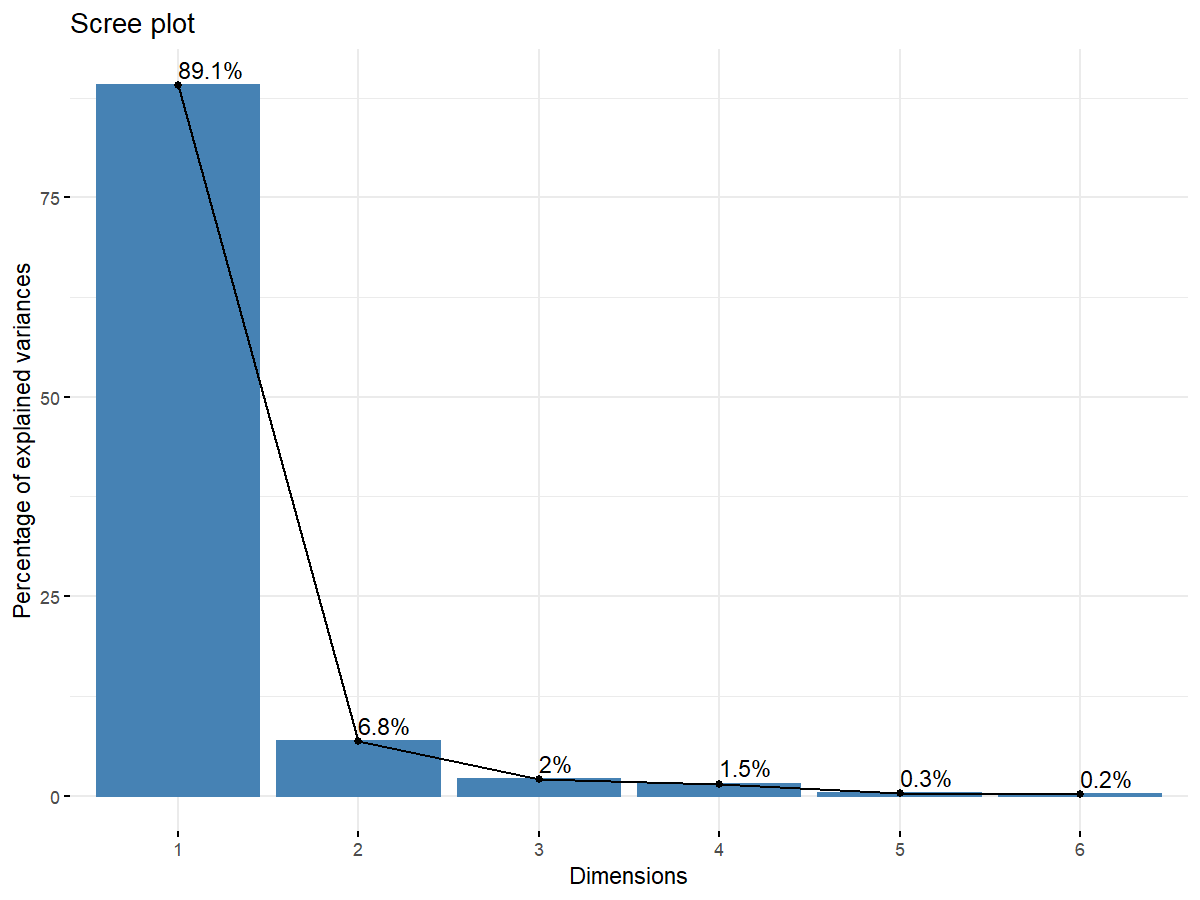Cool!

Finally, I'll use a different factoextra plotting method to show the PC scores in two different dimensions separated by my discrete factor, ecomorph.

``````fviz_pca_ind(obj,label="none",habillage=ecomorph,
``````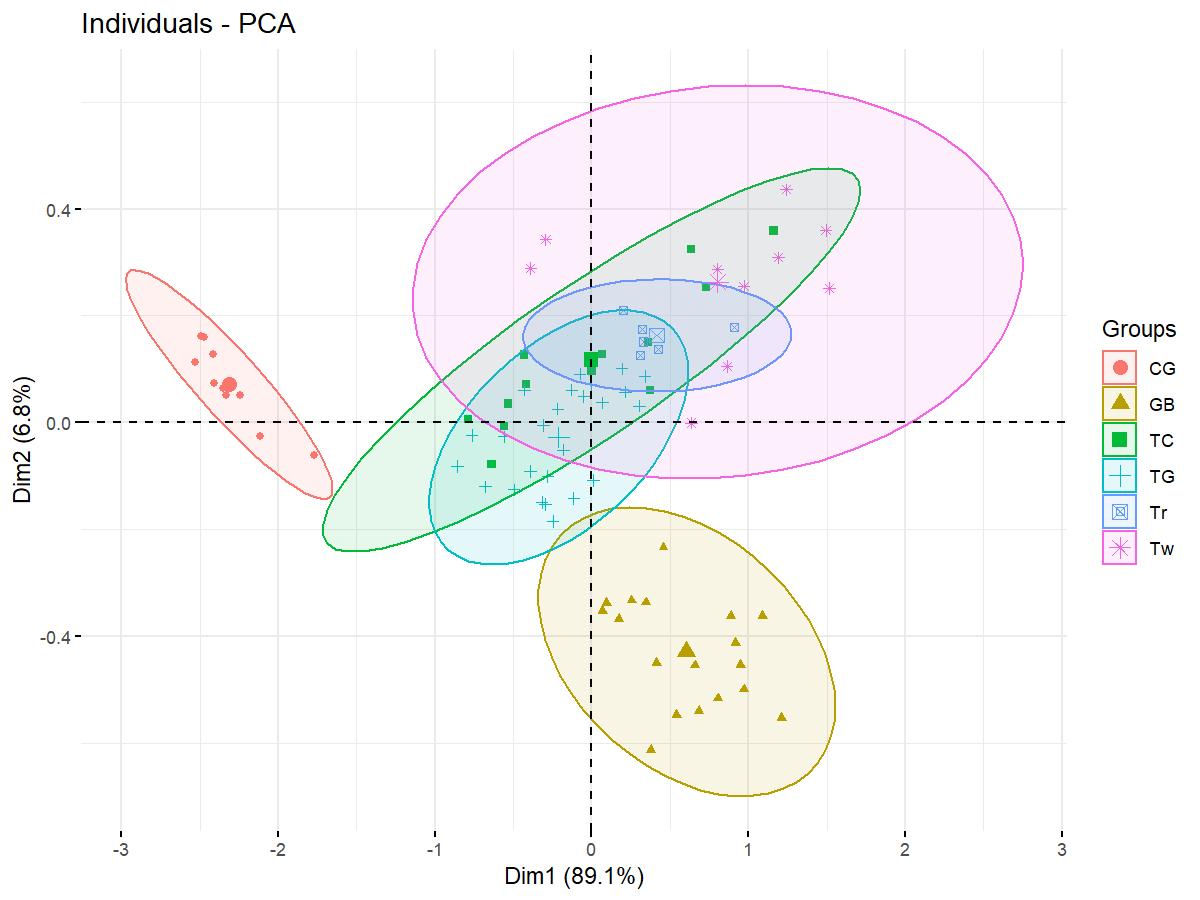That's it.

## Friday, March 11, 2022

### Plotting the posterior densities for ancestral node states from phytools `anc.Bayes`

Working with a technician in my lab yesterday (Viviana Romero Alarcon), I was reminded of the phytools function `anc.Bayes`, which does ancestral state estimation for continuous characters using Bayesian MCMC.

Although the point estimates from `anc.Bayes` will tend to be the same as ML (that is, assuming we use a relatively uninformative prior on node states – the default is designed to be quite uninformative), one cool feature of `anc.Bayes` is that it makes it pretty easy for us to measure uncertainty of ancestral state estimates by computing or plotting the posterior density for each node.

Here's a quick demo on how to do this using a data set for mammalian body size evolution from Garland et al. (1992).

``````## load packages and data
library(phytools)
data(mammal.data)
data(mammal.tree)
## extract body mass on a log scale
lnBodyMass<-setNames(log(mammal.data\$bodyMass),rownames(mammal.data))
## run MCMC
mcmc<-anc.Bayes(mammal.tree,lnBodyMass,ngen=1e6)
``````
``````## Control parameters (set by user or default):
``````
``````## List of 7
##  \$ sig2   : num 0.0796
##  \$ a      : num [1, 1] 4.62
##  \$ y      : num [1:47] 4.62 4.62 4.62 4.62 4.62 ...
##  \$ pr.mean: num [1:49] 1000 0 0 0 0 0 0 0 0 0 ...
##  \$ pr.var : num [1:49] 1e+06 1e+03 1e+03 1e+03 1e+03 1e+03 1e+03 1e+03 1e+03 1e+03 ...
##  \$ prop   : num [1:49] 0.0557 0.0557 0.0557 0.0557 0.0557 ...
##  \$ sample : num 100
``````
``````## Starting MCMC...
``````
``````## Done MCMC.
``````
``````## put the node indices into a vector
nodes<-as.character(1:mammal.tree\$Nnode+Ntip(mammal.tree))
## compute posterior densities for each node
pd<-lapply(nodes,function(nn,mcmc) density(mcmc,what=nn),mcmc=mcmc)
## get the full x and y ranges for the densities
xlim<-exp(range(sapply(pd,function(dd) range(dd\$x))))
ylim<-range(sapply(pd,function(dd) range(dd\$y)))
## plot all the densities
par(mar=c(5.1,4.1,3.1,1.1))
plot(NA,xlim=xlim,ylim=ylim,bty="n",ylab="density",xlab="body mass (kg)",
las=1,log="x")
clip(xlim,xlim,ylim,ylim)
grid()
cols<-colorRampPalette(colors=c("blue","red"))(mammal.tree\$Nnode)
nulo<-mapply(function(dd,col) polygon(exp(dd\$x),dd\$y,
col=make.transparent(col,0.2),border=col),dd=pd,col=cols)
title(main="posterior probability density from MCMC",font.main=3)
``````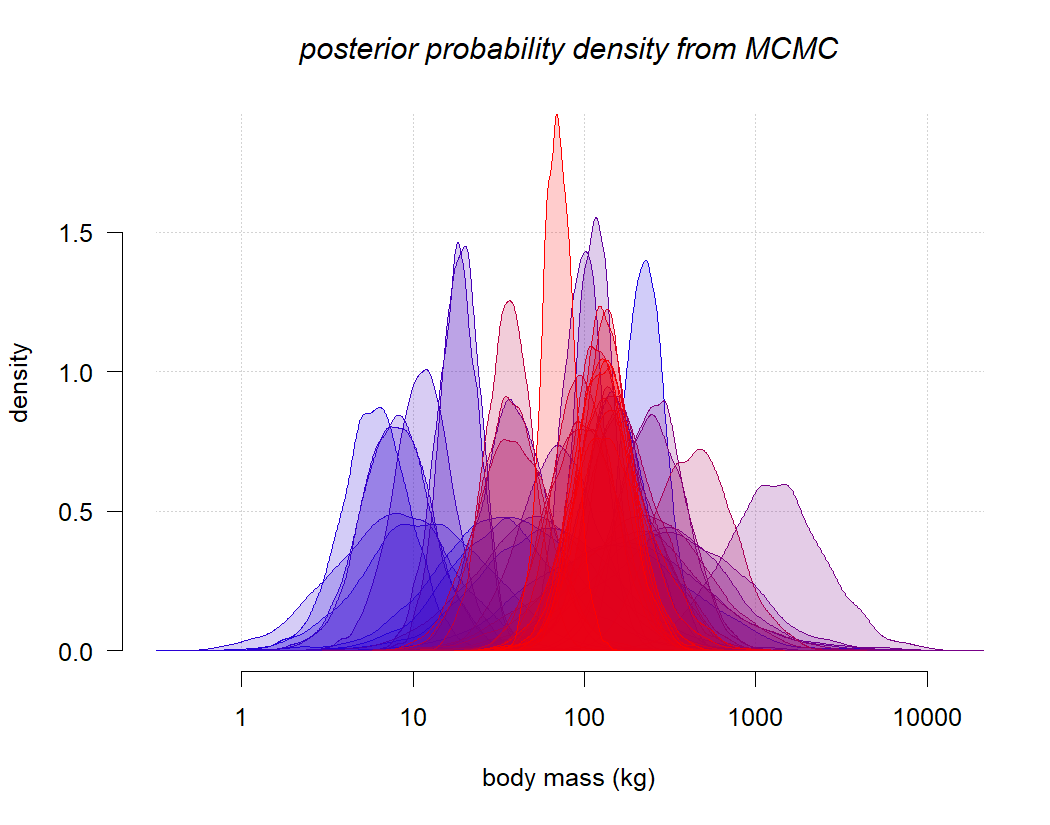Obviously, this is far too much to absorb in a single plot, but let's graph the color scheme used for the densities onto the nodes of the tree as follows:

``````plotTree(mammal.tree,ftype="i",fsize=0.8)
pp<-get("last_plot.phylo",envir=.PlotPhyloEnv)
points(pp\$xx[1:pp\$Nnode+pp\$Ntip],pp\$yy[1:pp\$Nnode+pp\$Ntip],pch=21,
col=cols,bg="white",cex=1.5)
points(pp\$xx[1:pp\$Nnode+pp\$Ntip],pp\$yy[1:pp\$Nnode+pp\$Ntip],pch=21,
col=cols,bg=sapply(cols,make.transparent,0.2),cex=1.5)
``````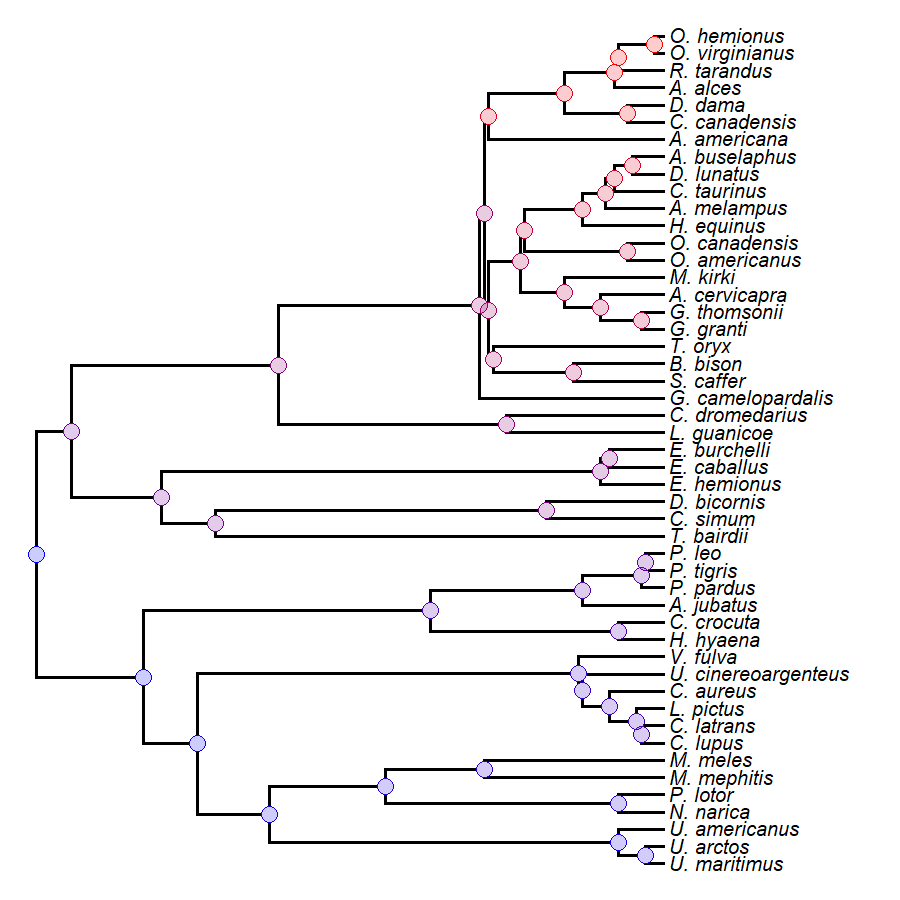Now, let's combine the two plots together using `layout`.

``````layout(matrix(c(2,1),1,2),widths=c(0.3,0.7))
par(mar=c(5.1,4.1,3.1,1.1))
plot(NA,xlim=xlim,ylim=ylim,bty="n",ylab="density",xlab="body mass (kg)",
las=1,log="x")
clip(xlim,xlim,ylim,ylim)
grid()
cols<-colorRampPalette(colors=c("blue","red"))(mammal.tree\$Nnode)
nulo<-mapply(function(dd,col) polygon(exp(dd\$x),dd\$y,
col=make.transparent(col,0.2),border=col),dd=pd,col=cols)
title(main="posterior probability density from MCMC",font.main=3)
plotTree(mammal.tree,ftype="i",fsize=0.6,mar=c(4.1,0.1,0.1,0.1))
pp<-get("last_plot.phylo",envir=.PlotPhyloEnv)
points(pp\$xx[1:pp\$Nnode+pp\$Ntip],pp\$yy[1:pp\$Nnode+pp\$Ntip],pch=21,
col=cols,bg="white",cex=1.5)
points(pp\$xx[1:pp\$Nnode+pp\$Ntip],pp\$yy[1:pp\$Nnode+pp\$Ntip],pch=21,
col=cols,bg=sapply(cols,make.transparent,0.2),cex=1.5)
``````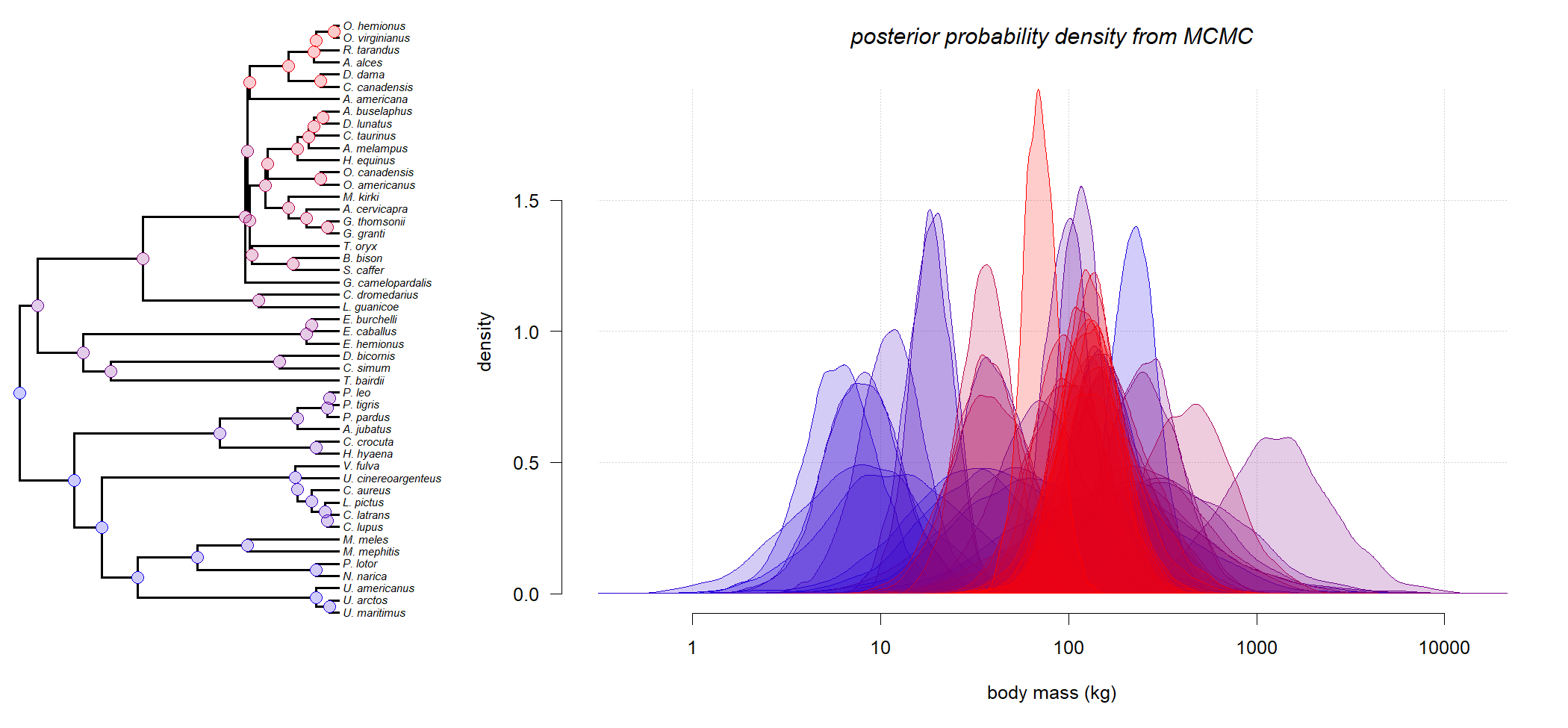That's pretty cool.

Now, let's try the same, but focusing on only a few different nodes in the tree.

``````plotTree(mammal.tree,ftype="i",fsize=0.7,lwd=1)
nodelabels(bg="white",cex=0.6,frame="circle")
``````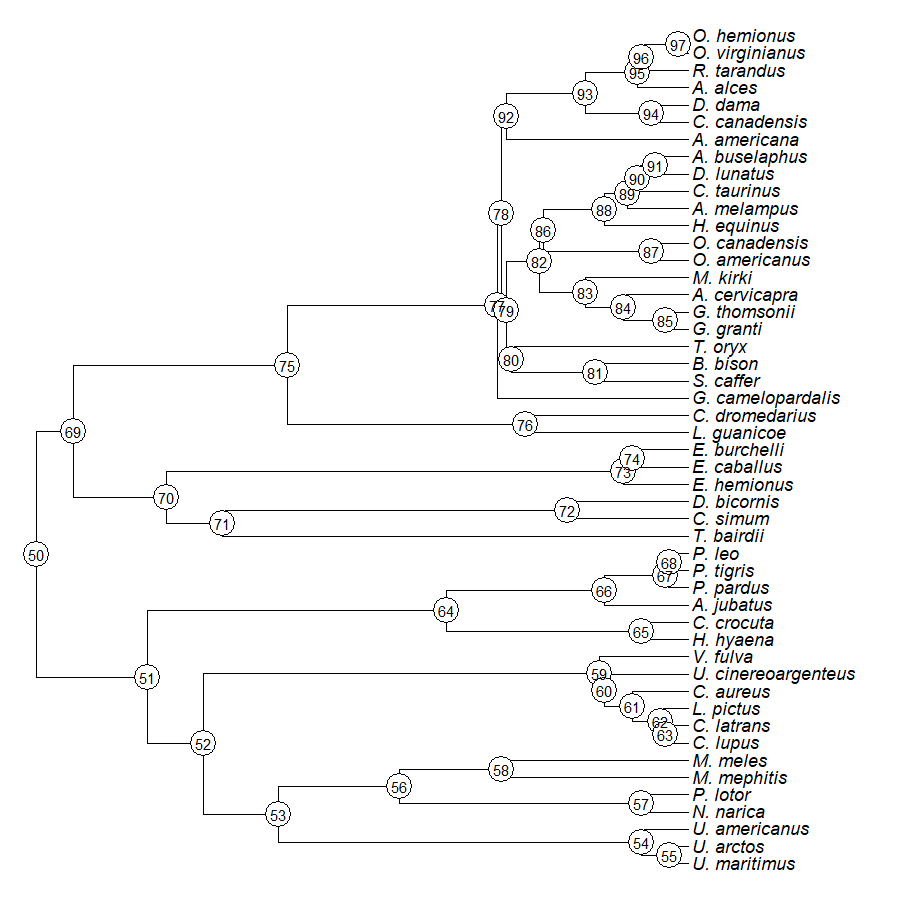We can pull out the posterior densities for the nodes corresponding to 'Carnivora' (node 51), 'Mustelidae' (node 58), 'Canidae' (node 59), 'Felidae' (node 66), 'Perrisodactyla' (node 70), 'Artiodactyla' (node 75), and 'Cervidae' (node 93).

``````nodes<-setNames(as.character(c(51,58,59,66,70,75,93)),
c('Carnivora','Mustelidae','Canidae','Felidae',
'Perrisodactyla','Artiodactyla','Cervidae'))
pd<-lapply(nodes,function(nn,mcmc) density(mcmc,what=nn),mcmc=mcmc)
xlim<-exp(range(sapply(pd,function(dd) range(dd\$x))))
ylim<-range(sapply(pd,function(dd) range(dd\$y)))
``````
``````## \$Carnivora
##
## Call:
##  density.anc.Bayes(x = mcmc, what = nn)
##
## Data: node 51 (8001 obs.);   Bandwidth 'bw' = 0.1353
##
##        x                y
##  Min.   :0.6908   Min.   :0.0000042
##  1st Qu.:2.4546   1st Qu.:0.0081821
##  Median :4.2184   Median :0.0615879
##  Mean   :4.2184   Mean   :0.1416008
##  3rd Qu.:5.9822   3rd Qu.:0.2783272
##  Max.   :7.7460   Max.   :0.4390239
##
## \$Mustelidae
##
## Call:
##  density.anc.Bayes(x = mcmc, what = nn)
##
## Data: node 58 (8001 obs.);   Bandwidth 'bw' = 0.1203
##
##        x                 y
##  Min.   :-0.9383   Min.   :0.0000048
##  1st Qu.: 0.5489   1st Qu.:0.0126695
##  Median : 2.0362   Median :0.0923058
##  Mean   : 2.0362   Mean   :0.1679297
##  3rd Qu.: 3.5234   3rd Qu.:0.3120443
##  Max.   : 5.0107   Max.   :0.4915038
``````

This time, we'll use a qualitative color palette from RColorBrewer instead of the gradient we used before:

``````cols<-brewer.pal(length(nodes),"Dark2")
par(mar=c(5.1,4.1,3.1,1.1))
plot(NA,xlim=xlim,ylim=ylim,bty="n",ylab="density",xlab="body mass (kg)",
las=1,log="x")
clip(xlim,xlim,ylim,ylim)
grid()
nulo<-mapply(function(dd,col) polygon(exp(dd\$x),dd\$y,
col=make.transparent(col,0.2),border=col),dd=pd,col=cols)
title(main="posterior probability density from MCMC",font.main=3)
legend("topright",names(nodes),pch=22,col=cols,pt.cex=1.5,
pt.bg=sapply(cols,make.transparent,0.2))
``````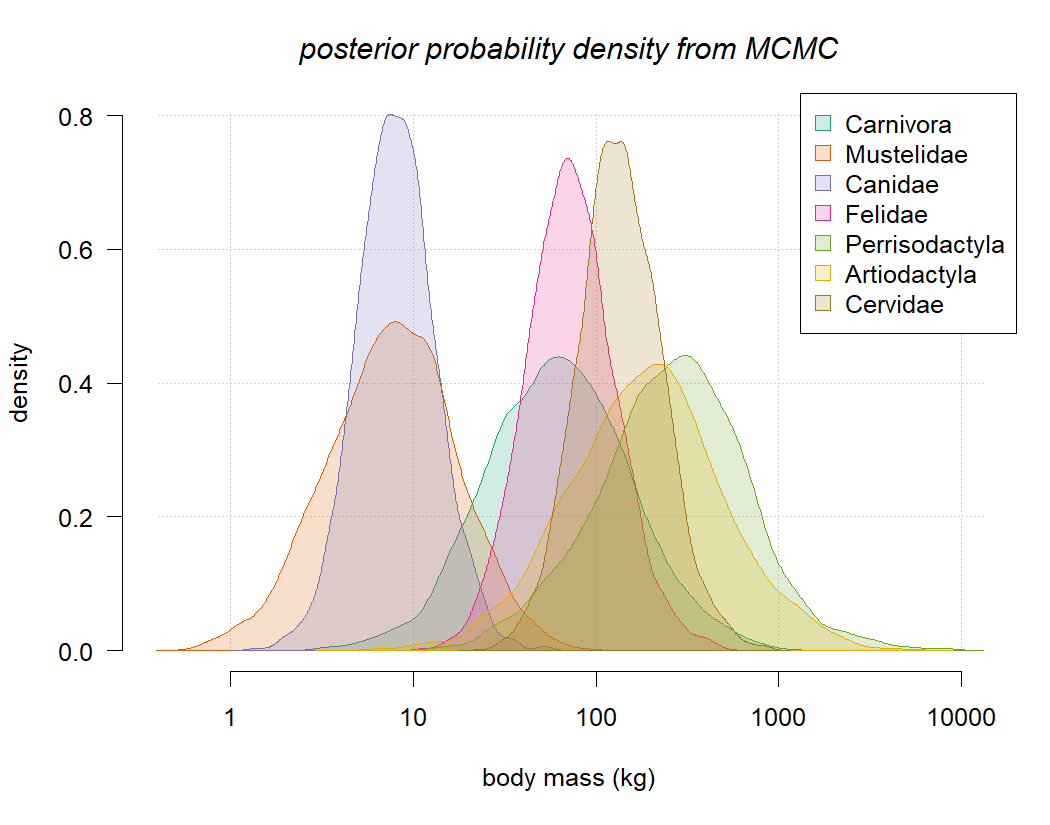Finally, let's plot it with our tree:

``````layout(matrix(c(2,1),1,2),widths=c(0.3,0.7))
par(mar=c(5.1,4.1,3.1,1.1))
plot(NA,xlim=xlim,ylim=ylim,bty="n",ylab="density",xlab="body mass (kg)",
las=1,log="x")
clip(xlim,xlim,ylim,ylim)
grid()
nulo<-mapply(function(dd,col) polygon(exp(dd\$x),dd\$y,
col=make.transparent(col,0.2),border=col),dd=pd,col=cols)
title(main="posterior probability density from MCMC",font.main=3)
legend("topright",names(nodes),pch=22,col=cols,pt.cex=1.5,
pt.bg=sapply(cols,make.transparent,0.2))
plotTree(mammal.tree,ftype="i",fsize=0.6,mar=c(4.1,0.1,0.1,0.1))
pp<-get("last_plot.phylo",envir=.PlotPhyloEnv)
points(pp\$xx[as.numeric(nodes)],pp\$yy[as.numeric(nodes)],pch=21,
col=cols,bg="white",cex=1.5)
points(pp\$xx[as.numeric(nodes)],pp\$yy[as.numeric(nodes)],pch=21,
col=cols,bg=sapply(cols,make.transparent,0.2),cex=1.5)
``````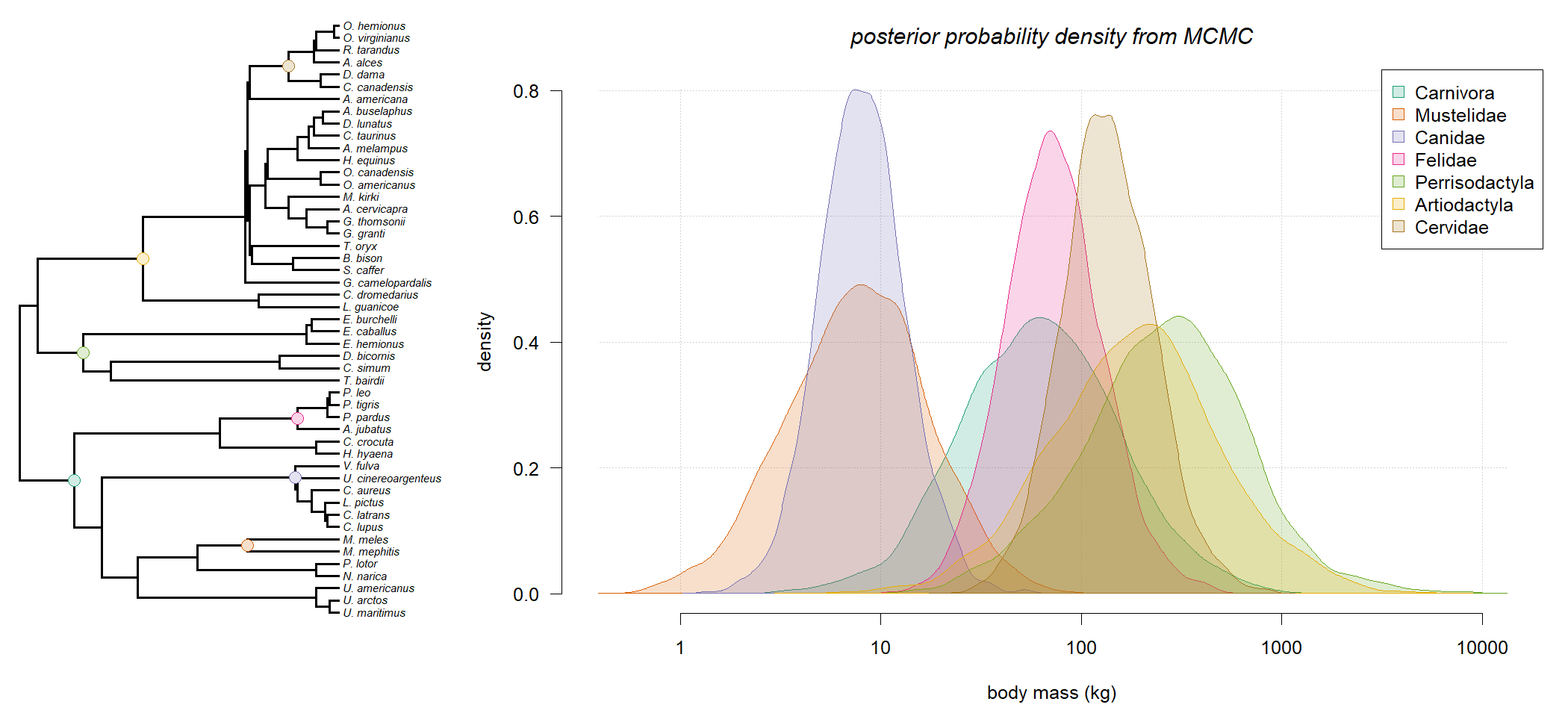I should caution, of course, that the specific values obtained here could certainly be biased by the specific taxa included in each group – which, in some cases (e.g., Felidae) tend to be much larger than the average for the group.